# seapy.subsystems.subsystem.Subsystem¶

class seapy.subsystems.subsystem.Subsystem(name, system, **properties)[source]

Bases: seapy.base.Base

Abstract Base Class for subsystems.

__init__(name, system, **properties)[source]

Constructor.

Parameters: name (string) – Identifier component (SeaPy.components.Component) – Component

Methods

 __init__(name, system, **properties) Constructor. addExcitation(name, model, **properties) Add excitation to subsystem. Subsystem.df disable([couplings]) Disable this subsystem. enable([couplings]) Enable this subsystem. info([attributes]) Return dataframe. plot(quantity[, yscale]) Plot quantity.

Attributes

 SORT str(object=’‘) -> str average_frequency_spacing “ classname Name of class of the object. component conductance Conductance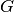. damping_term The damping term is the ratio of the modal half-power bandwidth to the average modal frequency spacing. dlf Damping loss factor of subsystem. enabled Switch indicating whether the object is enabled. energy Total energy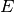in subsystem. frequency Frequency. impedance Impedance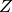included Indicates whether the object is included in the analysis. linked_couplings_from linked_couplings_to linked_excitations mobility Mobility Y modal_density Modal density. modal_energy Class capable of containing spectral values. modal_overlap_factor Modal overlap factor. name power_input Total input power due to excitations. resistance Resistance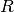, the real part of the impedance. soundspeed_group Group velocity in a subsystem. soundspeed_phase Phase velocity in a subsystem. tlf Total loss factor. wavenumber Wave number.
__init__(name, system, **properties)[source]

Constructor.

Parameters: name (string) – Identifier component (SeaPy.components.Component) – Component

average_frequency_spacing[source]

” Average frequency spacing.

component = None

Component this subsystem uses.

conductance[source]

Conductance.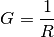damping_term[source]

The damping term is the ratio of the modal half-power bandwidth to the average modal frequency spacing.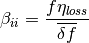See Lyon, above equation 12.1.4

disable(couplings=False)[source]

Disable this subsystem. Optionally disable dependent couplings as well.

Parameters: couplings (bool) – Disable couplings
dlf[source]

Damping loss factor of subsystem.

By default this is the loss factor of the material of the component.

enable(couplings=False)[source]

Enable this subsystem. Optionally enable dependent couplings as well.

Parameters: couplings (bool) – Enable couplings
energy[source]

Total energyin subsystem.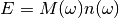impedance[source]

Impedancelinked_couplings_from = <generator object __get__ at 0x7f6dee211d38>

Set of couplings in which the subsystem is in the From field.

linked_couplings_to = <generator object __get__ at 0x7f6dee2111f8>

Set of couplings in which the subsystem is in the To field.

linked_excitations = <generator object __get__ at 0x7f6dee2115e8>

Set of excitations this subsystem experiences.

mobility[source]

Mobility Y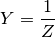modal_density[source]

Modal density.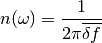See Lyon, eq. 8.1.6

modal_energy

Modal energy.

modal_overlap[source]

Modal overlap.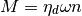with:

• damping loss factor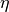• angular frequency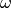• modal density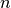See Craik, equation 2.23, page 41.

modal_overlap_factor[source]

Modal overlap factor.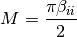See Lyon, above equation 12.1.4

power_input[source]

Total input power due to excitations.

resistance[source]

Resistance, the real part of the impedance.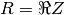soundspeed_group[source]

Group velocity in a subsystem.

soundspeed_phase[source]

Phase velocity in a subsystem.

tlf[source]

Total loss factor.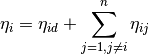See Craik, equation 3.18, page 60.

wavenumber[source]

Wave number.

#### Previous topic

seapy.subsystems.subsystemacoustical.SubsystemAcoustical

#### Next topic

seapy.subsystems.subsystemstructural.SubsystemStructural